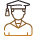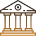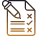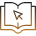# Real World Applications Of Matrices In Banking And Finance

###### ByRamraj Saini###### Synopsis

Matrices play a crucial role in banking and finance, enabling financial institutions to optimise portfolios, manage risks, and make informed decisions. This article explores the real-world applications of matrices in banking and finance, providing examples of how they are used to improve performance and minimise risks. For further insight, keep reading.###### Synopsis

Matrices play a crucial role in banking and finance, enabling financial institutions to optimise portfolios, manage risks, and make informed decisions. This article explores the real-world applications of matrices in banking and finance, providing examples of how they are used to improve performance and minimise risks. For further insight, keep reading.

Matrices are one of the most powerful mathematical tools used in various fields of science and engineering, and their applications in banking and finance are no exception. As Class 12 students gear up for a future in these industries, it's crucial to understand the real-world applications of matrices and how they contribute to the daily operations of banks and financial institutions.

In simple terms, matrices are a collection of numbers arranged in rows and columns. However, their usefulness lies in their ability to represent complex systems and processes that are integral to the functioning of financial institutions. From investment strategies to risk management, matrices play a significant role in the decision-making processes of banks and finance firms.

In this article, we will explore in-depth the various real-world applications of matrices in banking and finance, including portfolio optimisation, risk management, credit scoring, and fraud detection.

## Applications Of Matrices

In the world of finance, matrices are used to model and analyse complex financial systems, such as stock markets, banking systems, and investment portfolios. They allow financial professionals to understand the interdependencies and relationships between different assets, risks, and opportunities. With the help of matrices, analysts can make informed decisions about investments, portfolio management, and risk management.

### Risk Management

Risk management in banking and finance involves identifying, analysing, and managing the potential risks that financial institutions face. Matrices are a crucial tool in this process, allowing institutions to model and analyse various types of risks.

Credit risk, for example, refers to the risk of borrowers defaulting on their loans. Banks use matrices to analyse the creditworthiness of borrowers and to calculate the probability of default. One commonly used matrix is the credit migration matrix, which shows the movement of borrowers between credit ratings over time. This matrix helps banks to identify potential default risks and to develop strategies to mitigate those risks.

Market risk refers to the risk of losses due to changes in market conditions, such as changes in interest rates, exchange rates, or commodity prices. Banks use matrices to model and analyse market risk by calculating the covariance matrix of different assets. The covariance matrix shows the relationships between different assets and helps banks to develop strategies to minimise the impact of market fluctuations on their portfolios.

Operational risk refers to the risk of losses due to internal processes, systems, or people. Banks use matrices to analyse operational risk by calculating the correlation matrix of different processes, systems, or people. The correlation matrix shows the relationships between different operational risks and helps banks to identify potential vulnerabilities and to develop strategies to mitigate those risks.

Liquidity risk refers to the risk of a bank not having enough cash or liquid assets to meet its financial obligations. Banks use matrices to model and analyse liquidity risk by calculating the cash flow matrix. The cash flow matrix shows the expected inflows and outflows of cash over time and helps banks to identify potential liquidity shortfalls and to develop strategies to manage those risks

### Investment Analysis

Investment analysis is an important field that relies heavily on matrices to analyse the performance of various investment options. Matrices are powerful tools that help investors analyse the risk and return of investments and create investment portfolios that meet specific objectives.

One example of the use of matrices in investment analysis is the calculation of the expected return of a portfolio. To calculate the expected return, an investor needs to know the returns of each asset in the portfolio and the weight of each asset. The weight of an asset in a portfolio is the percentage of the portfolio's total value that is invested in that asset.

The expected return of a portfolio can be calculated using the following formula:

Expected return = (weight of asset 1 x return of asset 1) + (weight of asset 2 x return of asset 2) + ... + (weight of asset n x return of asset n)

For example, suppose an investor has a portfolio that consists of three assets:

 Assets Weight Expected Return Stock A 40% 10% Stock B 30% 8% Stock C 30% 5%

To calculate the expected return of the portfolio, the investor would use the following formula:

Expected return = (0.4 x 0.1) + (0.3 x 0.08) + (0.3 x 0.05) = 0.085 or 8.5%

Another example of the use of matrices in investment analysis is the calculation of the covariance matrix. The covariance matrix is a matrix that shows the covariance between each pair of assets in a portfolio. Covariance measures the degree to which the returns of two assets move together. A positive covariance means that the returns of two assets move together, while a negative covariance means that the returns of two assets move in opposite directions.

The covariance matrix can be calculated using the following formula:

Covariance matrix = Σ (weight of asset i x weight of asset j x covariance between asset i and asset j)

### Portfolio Optimisation

Portfolio optimisation is a technique used by investors to construct an investment portfolio that maximises returns while minimising risks. The process involves selecting a mix of assets, such as stocks, bonds, and commodities, that can provide the desired level of return while minimising risk. This can be accomplished by using matrices to calculate the optimal allocation of assets in the portfolio based on their expected returns, risks, and correlations.

One of the key benefits of portfolio optimization is that it enables investors to make informed decisions about their investments. Rather than simply choosing assets based on past performance or other subjective factors, investors can use quantitative analysis to identify the optimal mix of assets for their portfolio. This can help them to achieve their financial goals while minimising risks.

To illustrate how matrices can be used in portfolio optimization, let's consider a simple example. Suppose an investor is considering investing in two stocks, A and B. The investor expects stock A to have an average annual return of 10 per cent with a standard deviation of 15 per cent, while stock B is expected to have an average annual return of 12 per cent with a standard deviation of 20 per cent. The investor also knows that the correlation between the two stocks is 0.5.

To calculate the optimal allocation of assets in the portfolio, the investor can use a covariance matrix.

Covariance matrix = Σ (weight of asset i x weight of asset j x covariance between asset i and asset j)

Variance = σ2

Covariance matrix is given as

 σ2(A) σAσB * Correlation σBσA * Correlation σ2(B)

Var(A) = (15%)2 = 0.0225

Var(B) = (20%)2 = 0.04

Cov(A, B) = 15% x 20% x 0.5 = 0.015

Cov(B, A) = 20% x 15% x 0.5 = 0.015

In this case, the covariance matrix would be:

 Asset A B A 0.0225 0.015 B 0.015 0.04

The diagonal elements of the matrix represent the variances of each asset, while the off-diagonal elements represent the covariance between the assets.

Using this matrix, the investor can calculate the optimal allocation of assets in the portfolio by solving for the weights that minimise the portfolio variance subject to a target expected return. This can be accomplished using a mathematical optimization technique such as mean-variance optimisation.

Now you are aware of how Matrices play a crucial role in banking and finance, enabling financial institutions to optimise portfolios, manage risks, and make informed decisions.

## Careers360 helping shape your Career for a better tomorrow#### 250M+

Students#### 30,000+

Colleges#### 500+

ExamsE-Books